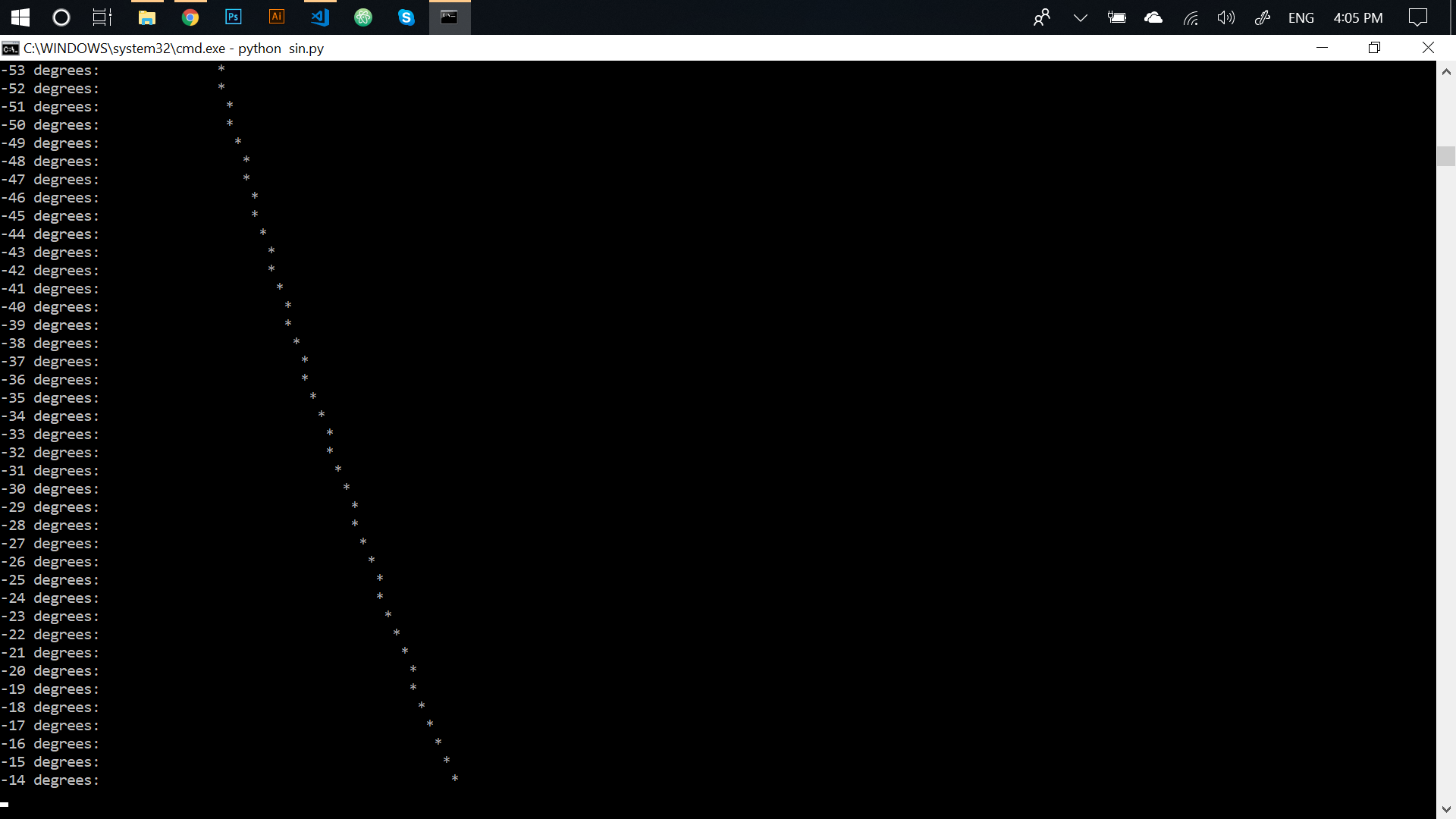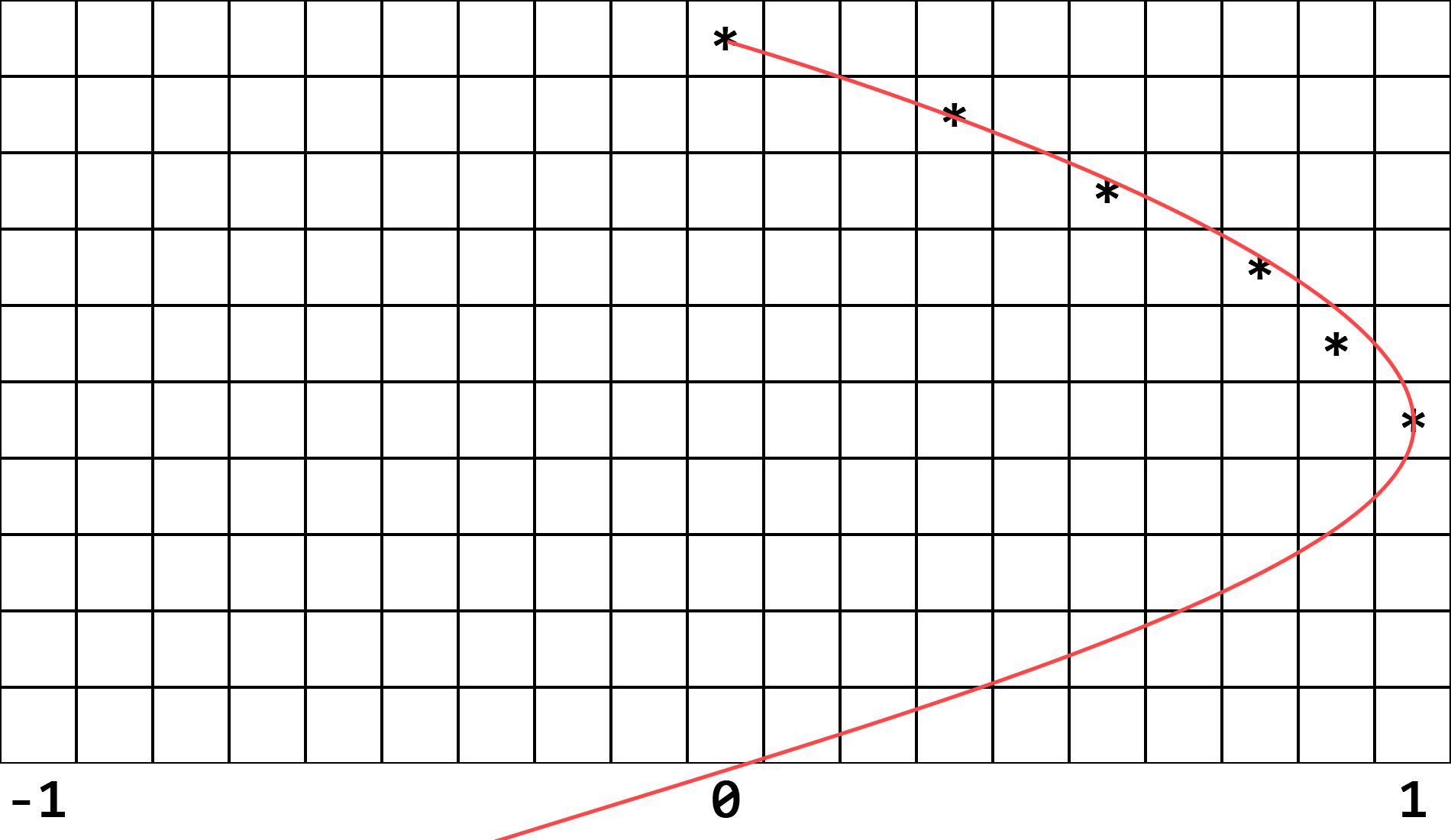Learn to Code via Tutorials on Repl.it!

← Back to all posts
Infinite Sine Wave in Python (really simple)

In my university they made engineers write a program in C (programming language) that prints out the sine wave. Many of my friends do engineering and they all told me about their "beloved" assignment.

It was about 8 p.m. and I was really bored... so I decided to do it myself but in Python (because I'm a lazy @ss). If you are interested, I am going to walk you through the process of this creation.

First of all, we have to `import` 3 useful functions from Python's standard library.

``````from math import sin, radians
from time import sleep``````
• `sin()` function returns the numerical (float) value of any angle in radians. We don't want to deal with those nasty radians (or at least I don't), so we import...
• ...`radians()` function that converts normal angles like 45°, 90°, 180° etc. into radians. As you may have guessed already, we are going to feed this value in radians to the `sin()` function.
• `sleep()` function allows us to slow down the printing of our sine wave by halting the program for a given amount of seconds. Computer calculates those values too fast and so we need to make it pause for a few milliseconds in order to see what it's printing.

Now that we have all those necessary functions, we can start coding for real. Python prints data into the Terminal line by line, so our graph will be vertical, top-to-bottom in direction.We also want our wave to be infinite so we are going to write a function `wave()` that prints out just one cycle of a sine wave -180 to 180 degrees and then we'll put it into the `while True` loop to make it repeat itself forever (or until you stop it by closing the Terminal window or pressing `Ctrl + C` known as Keyboard Interrupt). Therefore, this is the layout of our script:

``````from math import sin, radians
from time import sleep

def wave():

while True: # let the FUN last FOREVER
wave()``````

And we are almost there! Only 5 additional lines of code to go.

One cycle of our sine wave includes all the angles starting at -180° and finishing at 180°. All those angles will have to be processed one by one. When I say these words, they instantly remind me of a `for` loop and it is exactly what we need here.

``````# concentrating on the wave() function now:
def wave():
for a in range(-180, 180): # 'a' stands for 'angle'
# 1. process angle somehow
# 2. print angle at the beginning of line
# 3. print some spaces ending with *
# 4. sleep() for some time to slow down the printing``````

That's all we've got to do now. The first line is objectively the hardest bit here. It took me quite a bit of time to figure it out. Basically, our idea is that Terminal's space is divided into many lines and each line has many rectangles equal in size, each rectangle can hold one symbol; in our case we are only interested in spaces `_` and asterisks `*`.

Sine wave ranges from -1 to 1 on the `y` axis. We will set this graph's maximum value to be 100 rectangles wide excluding the angle value at the beginning of every line (it is going to be the rightmost asterisk in our graph). Its 0 value will be right in the middle of the line and its -1 value will be the leftmost rectangle.What we are really calculating here is the number of spaces in every line before the asterisk. This number of spaces increases and decreases with the sine value of the angle.

Long story short, let's write this line already.

Line 1: We've got to find the `sin()` for the angle `a` that is given by the `for` loop. This value we will store in a variable called `s` for `sine`.

``````def wave():
for a in range(-180, 180): # 'a' stands for 'angle'

Line 1: If we open the Terminal, run Python interpreter, import `sin()` and `radians()` functions, we can see the format of output.

``````Python 3.6.5 (v3.6.5:f59c0932b4, Mar 28 2018, 16:07:46) [MSC v.1900 32 bit (Intel)] on win32
>>> from math import sin, radians
0.7071067811865475``````

Line 1: We need to convert this value into an integer between 0 and 100 that is going to represent the number of spaces for each specific line. To do this, we multiply this ridiculous `float` by a 100 and display the result to 2 decimal places.

``````def wave():
for a in range(-180, 180): # 'a' stands for 'angle'
s = "{:.02f}".format( sin( radians(a) ) * 100 )``````

Line 1: Now, `s` is a `str` that looks like a `float`, but we want the `float` so we wrap all this into `float()` function.

``````def wave():
for a in range(-180, 180): # 'a' stands for 'angle'
s = float( "{:.02f}".format( sin( radians(a) ) * 100 ) )``````

Line 1: However, we actually need an integer, not a `float`, so we wrap it all again into the `round()` function.

``````def wave():
for a in range(-180, 180): # 'a' stands for 'angle'
s = round( float( "{:.02f}".format( sin( radians(a) ) * 100 ) ) )``````

Line 1: And the last thing for this line (I promise) is we need to divide this number by 2 because we have negative values as well, so both positive and negative side will be 50 spaces wide. Here we are, the first line is ready!

``````def wave():
for a in range(-180, 180): # 'a' stands for 'angle'
s = round( float( "{:.02f}".format( sin( radians(a) ) * 100 ) ) ) // 2``````

Now we simply need to use this value to print out the graph.

Line 2: First things first, we want to know what angle each line represents, so...

``````def wave():
for a in range(-180, 180): # 'a' stands for 'angle'
s = round( float( "{:.02f}".format( sin( radians(a) ) * 100 ) ) ) // 2
print(f"{a} degrees:", end="\t")
# for those who aren't familiar with this syntax
# this line is equivalent of
# print( "{} degrees:".format(a), end="\t" )``````

Line 3: Print `(s + 50)` spaces. The idea behind `s + 50` is that our `0` point must be in the middle and negative numbers must take up first 50 spaces, so we push every `s` by 50 spaces to the right. Note that negative numbers simply subtract from 50 and are therefore going to be placed left-of-center.

``````def wave():
for a in range(-180, 180):
s = round( float( "{:.02f}".format( sin( radians(a) ) * 100 ) ) ) // 2
print(f"{a} degrees:", end="\t")
print( (s + 50) * " ", end="*\n" )``````

Line 4: Last one and the easiest one. Just halt the process for 0.01 seconds to slow it down a bit.

``````def wave():
for a in range(-180, 180):
s = round( float( "{:.02f}".format( sin( radians(a) ) * 100 ) ) ) // 2
print(f"{a} degrees:", end="\t")
print( (s + 50) * " ", end="*\n" )
sleep(0.01)``````

Voila! Our `wave()` function is ready and the script is now complete.

minermaniac447 (250)

This is really cool, you get my upvote. I second @sharpvik's suggestion - either copypaste into your own repl or click 'open in Repl.It' on the top right of the embed.

CarolineZ (3)

This is really interesting, but is there a way where you can change the number of spaces so that it can fit into the screen, without making a flat line halfway through?

sharpvik (60)

@CarolineZ as I mentioned earlier, this program has not been written to be displayed in this small embedded terminal windown. Either open the repl itself and resize or download the file and open on your PC.

sharpvik (60)

Width of this embedded Terminal is not ideal for displaying the sine wave... better copy+paste and check it our on your computer in full-screen.